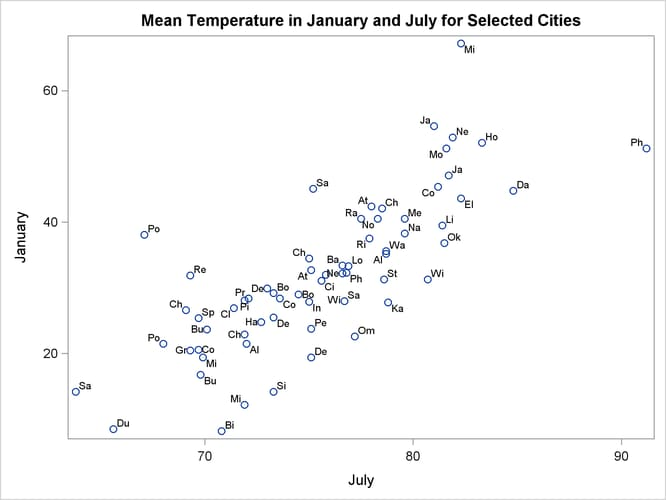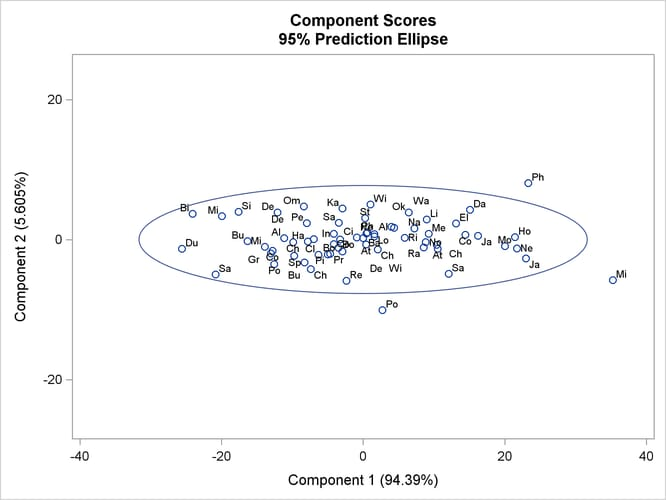# The PRINCOMP Procedure

### Example 73.1 Temperatures

This example analyzes mean daily temperatures in selected cities in January and July. Both the raw data and the principal components are plotted to illustrate how principal components are orthogonal rotations of the original variables.

The following statements create the `Temperature` data set.

```data Temperature;
length Cityid \$ 2;
title 'Mean Temperature in January and July for Selected Cities ';
input City \$1-15 January July;
Cityid = substr(City,1,2);
datalines;
Mobile          51.2 81.6
Phoenix         51.2 91.2
Little Rock     39.5 81.4
Sacramento      45.1 75.2
Denver          29.9 73.0

... more lines ...

Cheyenne        26.6 69.1
;
```

The following statements plot the `temperature` data set. The `Cityid` variable instead of `City` is used as a data label in the scatter plot for possible label clashing.

```title 'Mean Temperature in January and July for Selected Cities';
proc sgplot data=Temperature;
scatter x=July y=January / datalabel=Cityid;
run;
```

The results are displayed in Output 73.1.1, which shows a scatter diagram of the 64 pairs of data points with July temperatures plotted against January temperatures.

Output 73.1.1: Plot of Raw DataThe following step requests a principal component analysis on the `Temperature` data set:

```ods graphics on;

title 'Mean Temperature in January and July for Selected Cities';
proc princomp data=Temperature cov plots=score(ellipse);
var July January;
id Cityid;
run;
```

Output 73.1.2 displays the PROC PRINCOMP output. The standard deviation of `January` (11.712) is higher than the standard deviation of `July` (5.128). The COV option in the PROC PRINCOMP statement requests the principal components to be computed from the covariance matrix. The total variance is 163.474. The first principal component explains about 94% of the total variance, and the second principal component explains only about 6%. The eigenvalues sum to the total variance.

Note that `January` receives a higher loading on `Prin1` because it has a higher standard deviation than `July`, and the PRINCOMP procedure calculates the scores by using the centered variables rather than the standardized variables.

Output 73.1.2: Results of Principal Component Analysis

 Mean Temperature in January and July for Selected Cities

The PRINCOMP Procedure

 Observations 64 2

Simple Statistics
July January
Mean 75.60781250 32.09531250
StD 5.12761910 11.71243309

Covariance Matrix
July January
July 26.2924777 46.8282912
January 46.8282912 137.1810888

 Total Variance 163.474

Eigenvalues of the Covariance Matrix
Eigenvalue Difference Proportion Cumulative
1 154.310607 145.147647 0.9439 0.9439
2 9.162960   0.0561 1.0000

Eigenvectors
Prin1 Prin2
July 0.343532 0.939141
January 0.939141 -.343532

PLOTS=SCORE in the PROC PRINCOMP statement requests a plot of the second principal component against the first principal component as shown in Output 73.1.3. It is clear from this plot that the principal components are orthogonal rotations of the original variables and that the first principal component has a larger variance than the second principal component. In fact, the first component has a larger variance than either of the original variables `July` and `January`. The ellipse indicates that Miami, Phoenix, and Portland are possible outliers.

Output 73.1.3: Plot of Component 2 by Component 1